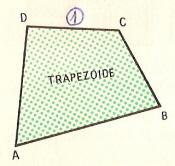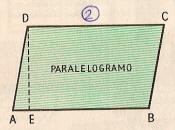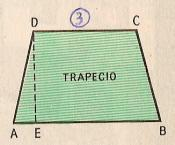Name: ______________________________________   Subject: _______________________  Date: _______

Write the missing word

1. Classification of quadrilaterals
A quadrilateral is a polygon with four sides.
In the figure 2, we see that its opposite sides are parallel. The side AB is parallel to DC. DA and CB are also parallel. This quadrilateral is called a parallelogram.
The figure 3 has two parallel sides: AB with CD. But the other two sides are not parallel. It is called trapeze, which is a quadrilateral with two opposite sides parallel.
The Figure 1 does not have any parallel side and it is called trapezoid.The Figure A is a...... The Figure B is a...... The Figure C is a......2. Classification of parallelograms

Parallelograms are quadrilaterals having opposite sides parallel. We can distinguish four classes:
1. The rhomboid: it has not right angles and the adjacent sides are unequal.
2. The rectangle: it has four right angles and the adjacent sides are not equal.
3. The rhombus: it has no right angles and the sides are equal.
4. The square: it has four right angles and their sides are equal. It is the regular quadrilateral.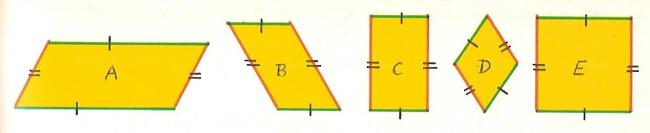Look at these pictures and answer these questions:

 The A is ... The C is ... The D is ... The B is ... The E is ...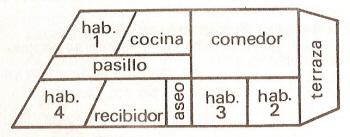3. Review
Observe the plan of this flat and answer:

 The room 1 is ... es... La cocina es... El comedor es... El pasillo es... El aseo es... The room 4 is ... es... El recibidor es... The room 2 is ... es... La tezarra es...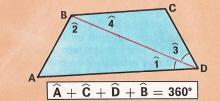4. Angle Sum of a quadrilateral

In the quadrilateral ABCD, we draw a diagonal BD and we get two triangles: BCD and ABD. We know that the sum of the angles of a triangle is 180º. We have two triangles and their angles will be 180° x 2 = 360°, that is, A + B + C + D = 360°.
The sum of the angles of a quadrilateral is 360° or four right angles.

| Educational applications | Mathematics |
In Spanish  | Interactive

®Arturo Ramo García.-Record of intellectual property of Teruel (Spain) No 141, of 29-IX-1999
Plaza Playa de Aro, 3, 1º DO 44002-TERUEL